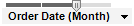﻿ Binning Functions

# Binning Functions

Note: If an axis using a custom expression with binning has been set up to Evaluate axis expression on: Current filtering only, then the binning will be recalculated with each filtering. See Details on Advanced Settings for more information.

 Function Description BinByDateTime Creates a binned column based on a natural date, datetime or time hierarchy. The first argument is the Date, Time or DateTime column to bin. The second argument is the definition of the levels in the hierarchy. The hierarchy levels should be written in the form of a string containing the desired date parts, separated by dots, for example "Year.Quarter.Month". The third argument is the pruning level which specifies the level of the hierarchy to display. If you write a custom expression based on a BinByDateTime expression you will see a column selector with all levels of the specified hierarchy available, but with the hierarchy slider handle positioned at the specified pruning level.   Valid arguments for Arg2 are combinations of: 'year' or 'yy' - The year. 'quarter' or 'qq' - The quarter. 'month' or 'mm' - The month. 'day of year' or 'dy' - The day of year. 'day' or 'dd' - The day. 'week' or 'wk' - The week. 'day of week' or 'dw' - The weekday. 'hour' or 'hh' - The hour. 'minute' or 'mi' - The minute. 'second' or 'ss' - The second. 'millisecond' or 'ms' - The millisecond.   Example: BinByDateTime([Column],"Year.Quarter.Month.Day",2)   For a date column called Order Date, the expression above would result in the column selector and hierarchy slider shown below:Pruning level 0 would set the slider handle to the year position, 1 would mean the quarter, 2 the month, and 3 the day. BinByEvenDistribution Creates a binned column where each bin has the same number of unique values as the others. The last bin may have more unique values than the others. The first argument is the column to bin and the second argument is the number of bins. Invalid values will give an invalid result.   Example: BinByEvenDistribution([Column], 5) BinByEvenDistribution(Rank([Column])*Count() + RowId(), 3) BinByEvenIntervals Creates a binned column where the value range is divided into equal intervals. The first argument is the column to bin and the second argument is the number of bins.   Example: BinByEvenIntervals([Column], 5) BinBySpecificLimits Creates a binned column with specific limits for the bins. The first argument is the column to bin and the following arguments are the limits for the bins. All rows which have values larger than the largest limit will have the same bin value. Invalid values will give an invalid result.   Example: BinBySpecificLimits([Column], 1, 2, 3, 10) BinByStdDev Creates a binned column where the values are divided into bins depending on the values distance from the mean, measured in standard deviations. The first argument is the column to bin and the following arguments are the number of standard deviations to show from the mean. The standard deviation arguments should be given in ascending order and all values should be positive.   Example: BinByStdDev([Column], 0.5, 1) This will create a binning for: ≤ -1 standard deviation -1 standard deviation -0.5 standard deviation 0.5 standard deviation 1 standard deviation > 1 standard deviation BinBySubstring Creates a binned column based on beginning or end of value. The first argument is the string column to bin and the following is the number of characters in the substring. If the second argument is negative the substring starts from the end of the value.   Examples: BinBySubstring([Column], -4) BinBySubstring(String([Integer Column]), 1) FiscalBinByDateTime Creates a binned column based on a natural date or datetime hierarchy, with the levels shifted the specified number of months.   The first argument is the Date or DateTime column to bin. The second argument is the hierarchy level definition written in the form of a string containing the desired date parts, separated by dots (e.g., "Year.Quarter.Month"). Only Year, Quarter and Month are supported. The third argument is the pruning level which specifies the level of the hierarchy to display. The fourth argument (optional) is the number of months to shift.   If no fourth argument is specified then the value of the document property FiscalYearOffset will be used.   Examples: FiscalBinByDateTime([Date Column], "Year.Quarter.Month", 1, 2) FiscalBinByDateTime([Date Column], "Year.Quarter.Month", 2)

Conversion functions

Date and Time functions

Logical functions

Math functions

OVER functions

Property functions

Ranking functions

Spatial functions

Statistical functions

Text functions